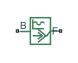# Sinusoidal Force Source

Produce sinusoidal force

•Libraries:
Simscape / Driveline / Sources

## Description

The Sinusoidal Force Source block produces sinusoidal force with a constant offset. A positive force value generates force acting from port B to port F.

The defining equation for the sinusoidal force that the block generates is

`$F={F}_{o}+A*\mathrm{sin}\left(2\pi ft+\phi \right),$`

where:

• F is the output force.

• Fo is the offset force of the output.

• A is the peak amplitude of the sinusoid.

• f is frequency of the sinusoid in Hz.

• t is time.

• φ is phase shift of the sinusoid.

## Ports

### Conserving

expand all

Mechanical translational conserving port associated with the base interface.

Mechanical translational conserving port associated with the follower interface.

## Parameters

expand all

Average value that the output sinusoidal force oscillates about.

Peak amplitude of the output sinusoidal force.

Phase shift of the output sinusoidal force.

Frequency of the output sinusoidal force. The frequency must be greater than or equal to 0.

## Version History

Introduced in R2016b